## Maximum-entropy module

In maxent.f90, all subroutines and functions are contained within the maxent module. The basis functions are computed in function phimaxent(), where Eq. (4) is used. To this end, the Lagrange multipliers are determined using the convex optimization algorithms that are presented in Section 2. Most of the functions and subroutines in this module are self-explanatory. We elaborate on the computation of the gradient of, and also on the Hessian, which is required in the Newton method. On taking the gradient (with respect to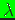) of, we obtain the negative of the left-hand side of the linear reproducing conditions in Eq. (3c):(6)

where(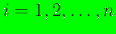). This is coded in function dfunc(). The expressions for the Hessian ofare given in Reference  (matrix form) as well as in the Appendix of Reference . By definition, the components of the Hessian are:(7)

The Hessian matrix (see Eq. (45) in Reference ) in three dimensions () is:(8)

whereis the expectation operator, which for a scalar-valued functionis: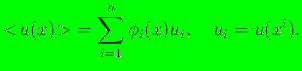(9)

The Hessian matrix and its inverse are computed in function hessian(flag) (flag is an optional Boolean argument) and function invhessian(flag), respectively.

Letdenote the converged solution for the Lagrange multipliers and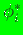the corresponding basis function solution for theth node. Since(-), the Hessian (hessian(.true.) is the call) is(10)

The expressions for the derivatives of the basis functions are given below. We adopt the notations and approach presented in Arroyo and Ortiz ; in the interest of space, just the final results are indicated. We can write Eq. (4) as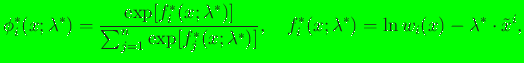(11)

whereis implicitly dependent on. On using Eq. (11), we haveand therefore the gradient ofis(13)

If the prior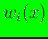is a Gaussian radial basis function (see Reference ), thenand Eq. (13) reduces to! This result appears in the Appendix of Reference . In general,depends onthrough the expression given in Eq. (13). The gradient of the basis functions is computed in function dphimaxent().

On taking the gradient of Eq. (13), we obtain the following expression for the second derivatives of the max-ent basis functions:(14)

where on using the identity, the gradient of the inverse of the Hessian can be written as(15)

and therefore(16)

The termin Eq. (14) is given by(17)

and the term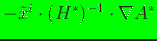is:The Hessian of the basis functions is computed in function ddphimaxent(). It satisfies the conditionsand.

N. Sukumar